ProGAN 是 ICLR 2018 提出的，并用于生成全高清(full-HD)照片逼真的图像，或平滑地组合任何先前生成的图像.

ProGAN 主要的四个创新点：

 - 渐进式增长和高分辨率层的平滑衰减(progressively growing and smoothly fading in higher resolution layers)

 - 小批量标准偏差(minibatch standard deviation)

 - 均衡学习率(equalized learning rate)

 - 逐像素特征归一化(pixel-wise feature normalization)

## 1. 隐空间插值(Latent space interpolation)## 2. ProGAN 的四个创新点

### 2.1. 渐进式增长和高分辨率层的平滑衰减

progressively growing and smoothly fading in higher resolution layers.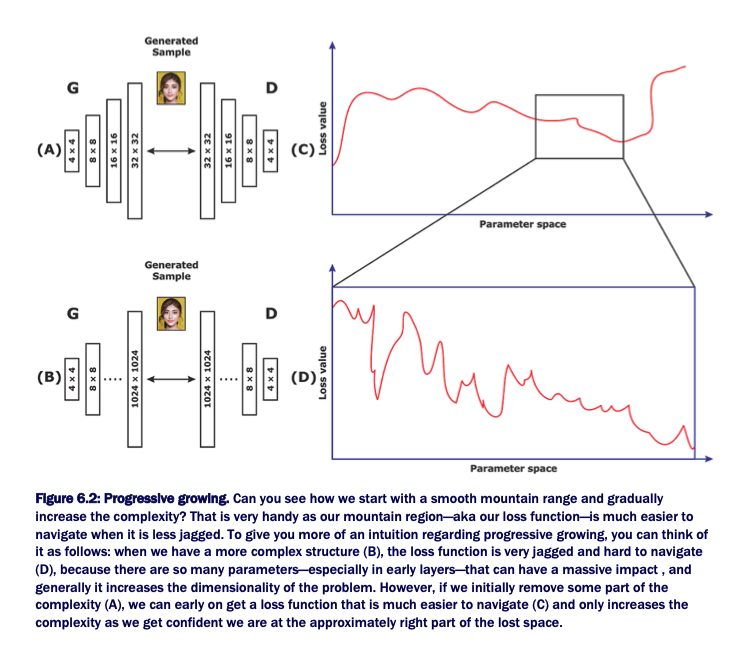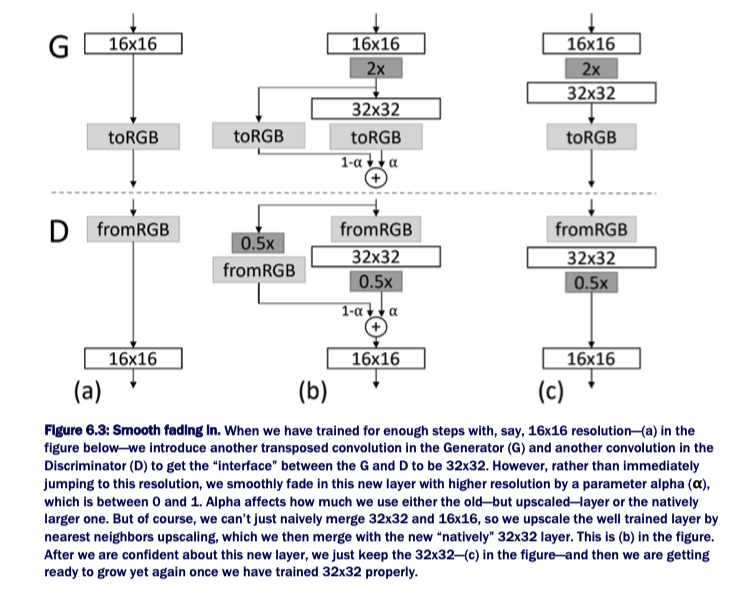import tensorflow as tf
import keras as K

#渐进式平滑(progressive smoothing)类似于:
def upscale_layer(layer, upscale_factor):
'''
Upscales layer (tensor) by the factor (int) where
the tensor is [group, height, width, channels]
'''
height = layer.get_shape()
width = layer.get_shape()
size = (scale * height, scale * width)
upscaled_layer = tf.image.resize_nearest_neighbor(layer, size)
return upscaled_layer

def smoothly_merge_last_layer(list_of_layers, alpha):
'''
Smoothly merges in a layer based on a threshold value alpha.
This function assumes: that all layers are already in RGB.
This is the function for the Generator.
:list_of_layers : items should be tensors ordered by size
:alpha : float \in (0, 1)
'''
# Hint!
# If you are using pure Tensorflow rather than keras, always remember scope
last_fully_trained_layer = list_of_layers
# now we have the originally trained layer
last_layer_upscaled = upscale_layer(last_fully_trained_layer, 2)

# this is the newly added layer not yet fully trained
larger_native_layer = list_of_layers[-1]

# This makes sure we can run the merging code
assert larger_native_layer.get_shape() == last_layer_upscaled.get_shape()

new_layer = (1-alpha) * upscaled_layer + larger_native_layer * alpha

return new_layer

### 2.2. 小批量标准偏差

minibatch standard devision.

 - 首先，计算 batch 内所有图像的标准偏差，以得到每个通道的每个像素的标准偏差所构成的单张图像.

 - 然后，计算所有通道的标准偏差，以得到单个特征图(feature map)或特定像素的标准偏差矩阵.

 - 最后，计算所有像素的标准偏差，以得到单个标量值.

def minibatch_std_layer(layer, group_size=4):
'''
Will calculate minibatch standard deviation for a layer.
Will do so under a pre-specified tf-scope with Keras.
Assumes layer is a float32 dat type. Else needs validation/casting.
Note: there is a more efficient way to do this in Keras, but just for
clarity and alignment with major implementations (for understanding)
this wad done more explicitly. Try thsi as an exercise.
'''
# Hint!
# If you are using pure Tensorflow rather than Keras, always remember scope
# minibatch group must be divisible by (or <=) group_size
group_size = K.backend.minimum(group_size, tf.shape(layer))

# just getting some shape information so that we can use
# them as shorthand as well as to ensure defaults
s = list(K.init_shape(int))
s = tf.shape(intput)

# Reshaping so that we operate on the level of hte minibatch
# in this code we assume the layer to be:
# [Group (G), Minibatch (M), Width (W), Height (H), channel (C)]
# but be careful different impelmentations use the Theano specific
minibatch = K.backend.reshape(layer, (group_size, -1, s, s, s))

# Center the mean over the group [M, W, H, C]
minibatch -= tf.reduce_mean(minibatch, axis=0, keepdims=True)
# Calculate the variance of the group [M, W, H, C]
minibatch = tf.reduce_mean(K.backend.square(minibatch), axis = 0)
# Calculate the standard deviation over the group [M, W, H, C]
minibatch = K.backend.square(minibatch + 1e8)
# Take average over feature maps and pixels [M, 1, 1, 1]
minibatch = tf.reduce_mean(minibatch, axis=[1,2,4], keepdims=True)
# Add as a layer for each group and pixels
minibatch = K.backend.tile(minibatch, [group_size, 1, s, s])
# Append as a new feature map
return K.backend.concatenate([layer, minibatch], axis=1)

### 2.3. 均衡化学习率

Equalized learning rate.

def equalize_learning_rate(shape, gain, fan_in=None):
'''
This adjusts the weights of every layer by the constant from He's initializer so
that we adjust for the variance in the dynamic range in different features
shape  : shape of tensor (layer): [kernel, kernel, height, feature_maps]
gain   : typically sqrt(2)
fan_in : adjustment for the number of incoming connections as per Xavier's / He's initialization
'''
# Default value is product of all the shape dimension minus the feature maps dim -- this gives us the number of incoming connections per neuron
if fan_in is None: fan_in = np.prod(shape[:-1])
# This uses He's initialization constant
std = gain / K.sqrt(fan_in)
# creates a constant out of the adjustment
wscale = K.constant(std, name='wscale', dtype=np.float32)
# gets values for weights and then uses broadcasting to apply the adjustment
initializer=tf.initializers.random_normal()) * wscale

return adjusted_weights

### 2.4. 逐像素特征归一化

Pixel-wise feature normalization.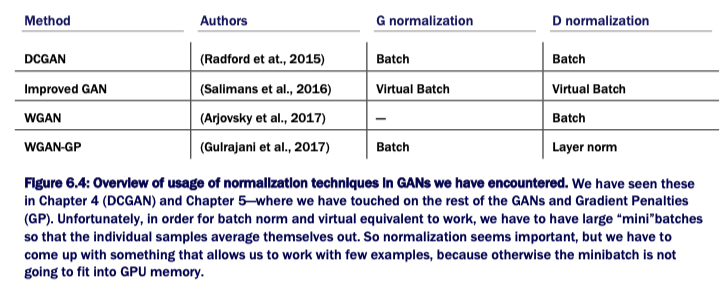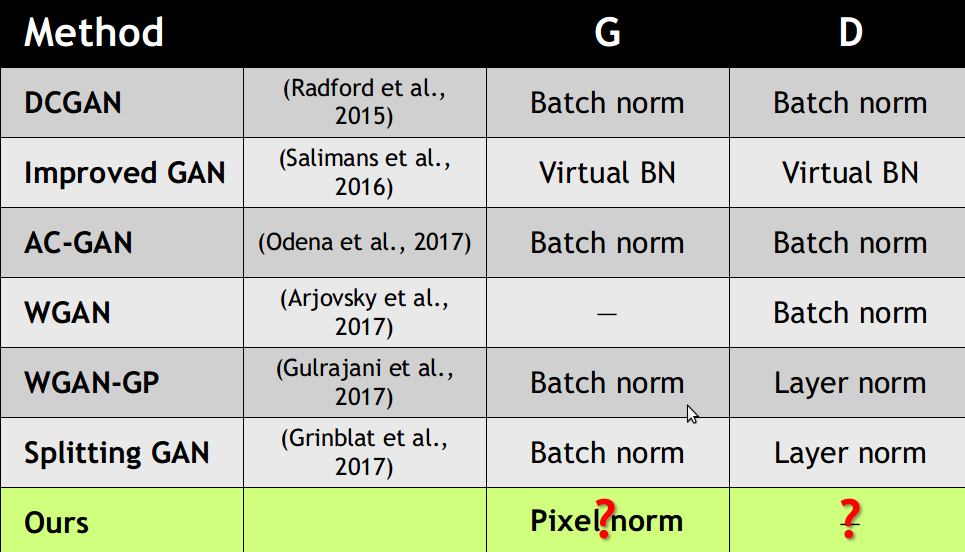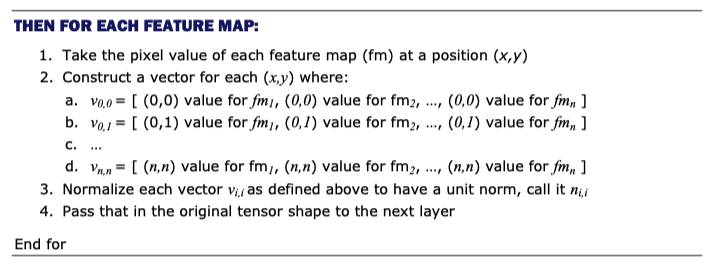$$n_{(x, y)} = \frac{a_{(x, y)}}{\sqrt{\frac{1}{N} \sum_{j=0}^{N-1} (a_{x, y}^j)^2 + \epsilon}}$$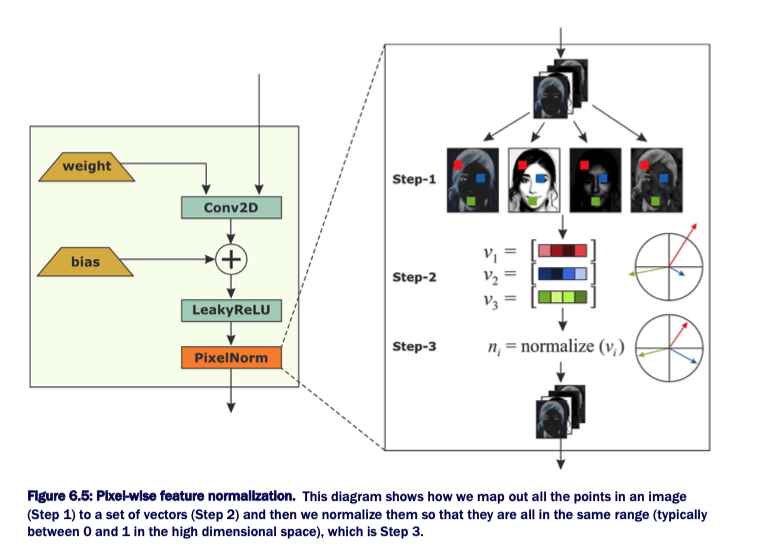def pixelwise_feat_norm(inputs, **kwargs):
'''
Uses pixelwise feature normalization as proposed by Krizhevsky et at. 2012.
Returns the input normalized
inputs : Keras / TF Layers
'''
normalization_constant = K.backend.sqrt(K.backend.mean(input**2, axis=-1, keepdims=True) + 1.0e-8)

return inputs / normalization_constant

## 参考

Last modification：September 3rd, 2020 at 03:47 pm